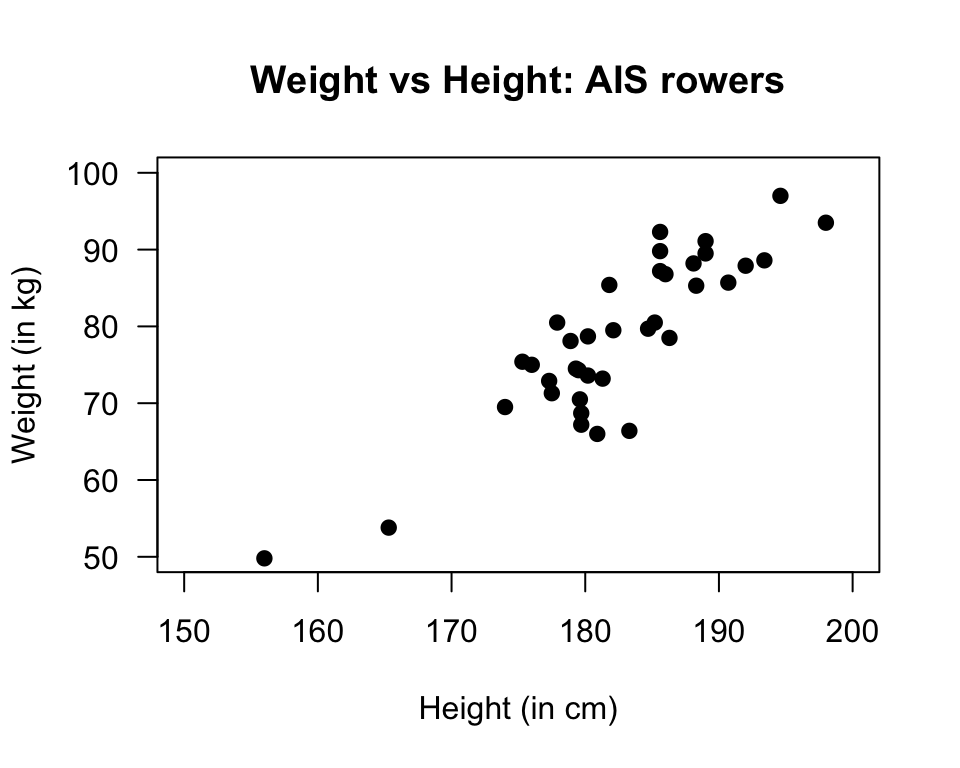## 35.12 Quick review questions

A study of athletes examined the relationship between the height and weight of $$n=37$$ rowers at the Australian Institute of Sport (AIS), as shown in Fig. 35.19.FIGURE 35.19: Scatterplot of Weight against Height rowers at the AIS

1. Using the ‘rise over run’ idea, the slope is approximately
2. The $$y$$-variable is
3. The regression equation is $$\hat{y} = -138 + 1.2 x$$. What does $$x$$ represent?
4. What does the ‘hat’ above the $$y$$ mean?
5. To two decimal places, what weight would be predicted for a rower who is 180cm tall?
6. The standard error of the slope is 0.112. What is the value of the test statistic (to one decimal place) to test if the population slope is zero?
7. True or false? The $$P$$-value for this test will be very small
8. True or false? The units of the slope are kg/cm
9. True or false? Making a prediction for the weight of a rower weighing 220 kg would be an example of extrapolation

### References

Telford RD, Cunningham RB. Sex, sport, and body-size dependency of hematology in highly trained athletes. Medicine and Science in Sports and Exercise. 1991;23(7):788–94.